Monday 18th January 2021

CBSE Guess > Papers > Question Papers > Class XII > 2003 > Mathematics > Outside Delhi Set -II

MATHEMATICS—2003 (Set II—Outside Delhi)

Note: Except for the following questions, all the remaining questions have been asked in Set I .

SECTION - A

Q. 3. Evaluate: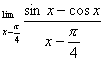Q. 7. Evaluate: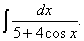Q. 10. Evaluate: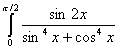Q. 14. Bag A contains 6 red and 5 blue balls and another bag B contains 5 red and 8 blue balls. A ball is drawn from the bag A without seeing its colour and it put into the bag B. then a ball is draw from bag B at random. Find the probability that eat the ball drawn is blue in colour.

Q. 18. Show that the height of a closed circular cylinder of given total surface areas and maximum volume is equal to the diameter of its base.

Q. 19. Given that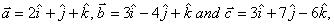verify that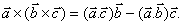Q. 23. Solve the following differential equation: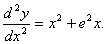SECTION - C

Q. 20. A firm knows that the demand function for one of its products is linear. It also known that it can sell 1000 units when the price is Rs. 4 per unit, and can sell 1500 units when the price is Rs. 2 per unit. Determine.
(i) The demand function,
(ii) The total revenue function,
(iii) The marginal revenue function.

 Mathematics 2003 Question Papers Class XII Delhi Outside Delhi Compartment Delhi Compartment Outside DelhiSet ISet ISet ISet ISet IISet IISet IISet IISet IIISet III

CBSE 2003 Question Papers Class XII